+关注继续查看

# 痛点：

1、就是买了 IaaS 却不会用
2、PaaS、SaaS 的自由度不高
3、ECS 附加功能太多还用不到，价格贵

1、轻量应用服务器 是 IaaS 产品但是提供定制化应用镜像且有可视化控制台可以操作，不怕新手不会用。
2、提供定制化应用镜像的同时，用户依旧可以拥有 root 权限，对系统进行个性化操作
3、精简了一些 ECS 的功能，比如说安骑士。把安全组的操作也做了适当减法更适合用户操作

轻量应用服务器 是 IaaS 产品但是提供定制化应用镜像且有可视化控制台可以操作，不怕新手不会用。轻量应用服务器 在提供提供定制化应用镜像的同时，用户依旧可以拥有 root 权限，对系统进行个性化操作精简了一些 ECS 的功能，比如说安骑士。把安全组的操作也做了适当减法更适合用户操作

# 功能

ECS 能就只有空白的系统镜像，任何环境都是需要用户自行安装的，这在一定程度上增加了用户使用的学习成本。而 ECS 更多体现的就是专业性了，虽然复杂但是十分强大的安全组、弹性IP、均衡负载等等。

# 缺点

Apache Httpd、PHP、MySQL 是会持续更新的，而且它们也均有爆发过大规模严重漏洞的历史，不过目前还没有看到应用镜像中的三大金刚如何升级版本号的姿势。

# CPU 性能

System Benchmarks Index Values               BASELINE       RESULT    INDEXDhrystone 2 using register variables         116700.0   34281977.0   2937.6Double-Precision Whetstone                       55.0       2962.7    538.7Execl Throughput                                 43.0       4860.0   1130.2File Copy 1024 bufsize 2000 maxblocks          3960.0    1054197.7   2662.1File Copy 256 bufsize 500 maxblocks            1655.0     283098.8   1710.6File Copy 4096 bufsize 8000 maxblocks          5800.0    3165160.0   5457.2Pipe Throughput                               12440.0    1734490.3   1394.3Pipe-based Context Switching                   4000.0     375732.0    939.3Process Creation                                126.0      18345.1   1456.0Shell Scripts (1 concurrent)                     42.4       6747.0   1591.3Shell Scripts (8 concurrent)                      6.0        921.5   1535.8System Call Overhead                          15000.0    2939229.7   1959.5
                                                               ========

System Benchmarks Index Score 1649.5

ECS：

System Benchmarks Index Values               BASELINE       RESULT    INDEXDhrystone 2 using register variables         116700.0   34582858.9   2963.4Double-Precision Whetstone                       55.0       2984.8    542.7Execl Throughput                                 43.0       5118.8   1190.4File Copy 1024 bufsize 2000 maxblocks          3960.0    1073823.9   2711.7File Copy 256 bufsize 500 maxblocks            1655.0     284701.5   1720.3File Copy 4096 bufsize 8000 maxblocks          5800.0    3213933.6   5541.3Pipe Throughput                               12440.0    1722785.7   1384.9Pipe-based Context Switching                   4000.0     377999.7    945.0Process Creation                                126.0      18704.4   1484.5Shell Scripts (1 concurrent)                     42.4       6852.6   1616.2Shell Scripts (8 concurrent)                      6.0        937.2   1562.0System Call Overhead                          15000.0    2979190.8   1986.1
                                                               ========

System Benchmarks Index Score 1673.4

# 磁盘性能

/dev/vda: Timing cached reads:   21070 MB in  2.00 seconds = 10549.25 MB/sec Timing buffered disk reads: 270 MB in  3.01 seconds =  89.76 MB/sec

[root@izbp1auqkyqtj4iozs7athz dev]# fio --bs=4k --ioengine=libaio --iodepth=1 --direct=1 --rw=read --time_based --runtime=600 --refill_buffers --norandommap --randrepeat=0 --group_reporting --name=fio-read --size=10G --filename=/dev/vdafio-read: (g=0): rw=read, bs=4K-4K/4K-4K/4K-4K, ioengine=libaio, iodepth=1fio-2.2.8Starting 1 processJobs: 1 (f=1): [R(1)] [100.0% done] [7200KB/0KB/0KB /s] [1800/0/0 iops] [eta 00m:00s]fio-read: (groupid=0, jobs=1): err= 0: pid=24361: Sun Sep 10 15:04:27 2017 read : io=4206.7MB, bw=7179.3KB/s, iops=1794, runt=600004msec

slat (usec): min=3, max=165, avg= 6.78, stdev= 2.02
clat (usec): min=2, max=84748, avg=548.56, stdev=2727.93
lat (usec): min=131, max=84755, avg=555.59, stdev=2727.93
clat percentiles (usec):
|  1.00th=[  141],  5.00th=[  241], 10.00th=[  302], 20.00th=[  306],
| 30.00th=[  314], 40.00th=[  322], 50.00th=[  334], 60.00th=[  350],
| 70.00th=[  362], 80.00th=[  382], 90.00th=[  442], 95.00th=[  502],
| 99.00th=[ 1012], 99.50th=, 99.90th=, 99.95th=,
| 99.99th=
bw (KB  /s): min= 4624, max= 7784, per=100.00%, avg=7182.85, stdev=181.15
lat (usec) : 4=0.01%, 250=6.25%, 500=88.69%, 750=3.79%, 1000=0.27%
lat (msec) : 2=0.36%, 4=0.06%, 10=0.03%, 20=0.04%, 50=0.48%
lat (msec) : 100=0.04%

cpu : usr=0.50%, sys=1.81%, ctx=1076903, majf=1, minf=33 IO depths : 1=100.0%, 2=0.0%, 4=0.0%, 8=0.0%, 16=0.0%, 32=0.0%, >=64=0.0%

 submit    : 0=0.0%, 4=100.0%, 8=0.0%, 16=0.0%, 32=0.0%, 64=0.0%, &gt;=64=0.0%
complete  : 0=0.0%, 4=100.0%, 8=0.0%, 16=0.0%, 32=0.0%, 64=0.0%, &gt;=64=0.0%
issued    : total=r=1076894/w=0/d=0, short=r=0/w=0/d=0, drop=r=0/w=0/d=0
latency   : target=0, window=0, percentile=100.00%, depth=1


Run status group 0 (all jobs): READ: io=4206.7MB, aggrb=7179KB/s, minb=7179KB/s, maxb=7179KB/s, mint=600004msec, maxt=600004msec

Disk stats (read/write): vda: ios=1078167/690, merge=0/399, ticks=598805/4230, in_queue=602927, util=98.01%

ECS：

/dev/vdb: Timing cached reads:   19778 MB in  2.00 seconds = 9901.01 MB/sec Timing buffered disk reads: 270 MB in  3.02 seconds =  89.54 MB/sec

[root@iZbp1258gr0v9v184jdqqkZ dev]# fio --bs=4k --ioengine=libaio --iodepth=1 --direct=1 --rw=read --time_based --runtime=600 --refill_buffers --norandommap --randrepeat=0 --group_reporting --name=fio-read --size=10G --filename=/dev/vdbfio-read: (g=0): rw=read, bs=4K-4K/4K-4K/4K-4K, ioengine=libaio, iodepth=1fio-2.2.8Starting 1 processJobs: 1 (f=1): [R(1)] [100.0% done] [6940KB/0KB/0KB /s] [1735/0/0 iops] [eta 00m:00s]fio-read: (groupid=0, jobs=1): err= 0: pid=9552: Sun Sep 10 15:23:44 2017 read : io=4218.9MB, bw=7200.2KB/s, iops=1800, runt=600001msec

slat (usec): min=3, max=344, avg= 6.97, stdev= 2.32
clat (usec): min=1, max=85711, avg=546.79, stdev=4741.30
lat (usec): min=145, max=85717, avg=553.99, stdev=4741.28
clat percentiles (usec):
|  1.00th=[  147],  5.00th=[  149], 10.00th=[  151], 20.00th=[  153],
| 30.00th=[  155], 40.00th=[  159], 50.00th=[  167], 60.00th=[  199],
| 70.00th=[  229], 80.00th=[  241], 90.00th=[  249], 95.00th=[  270],
| 99.00th=[  374], 99.50th=, 99.90th=, 99.95th=,
| 99.99th=
bw (KB  /s): min= 6380, max= 7624, per=100.00%, avg=7202.00, stdev=111.69
lat (usec) : 2=0.01%, 50=0.01%, 250=90.56%, 500=8.76%, 750=0.06%
lat (usec) : 1000=0.02%
lat (msec) : 2=0.02%, 4=0.01%, 10=0.01%, 20=0.01%, 50=0.01%
lat (msec) : 100=0.55%

cpu : usr=0.50%, sys=1.98%, ctx=1080029, majf=0, minf=34 IO depths : 1=100.0%, 2=0.0%, 4=0.0%, 8=0.0%, 16=0.0%, 32=0.0%, >=64=0.0%

 submit    : 0=0.0%, 4=100.0%, 8=0.0%, 16=0.0%, 32=0.0%, 64=0.0%, &gt;=64=0.0%
complete  : 0=0.0%, 4=100.0%, 8=0.0%, 16=0.0%, 32=0.0%, 64=0.0%, &gt;=64=0.0%
issued    : total=r=1080027/w=0/d=0, short=r=0/w=0/d=0, drop=r=0/w=0/d=0
latency   : target=0, window=0, percentile=100.00%, depth=1


Run status group 0 (all jobs): READ: io=4218.9MB, aggrb=7200KB/s, minb=7200KB/s, maxb=7200KB/s, mint=600001msec, maxt=600001msec

Disk stats (read/write): vdb: ios=1079640/0, merge=0/0, ticks=589523/0, in_queue=589382, util=98.27%

# 一：优惠价格表——阿里云轻量应用服务器价格表# 三：轻量应用服务器与ECS服务器性能比较

“轻量”二字，先入为主，让我们觉得是不是同等配置下的轻量服务器性能就一定不如ECS服务器呢？

### 阿里云服务器：活动地址0 0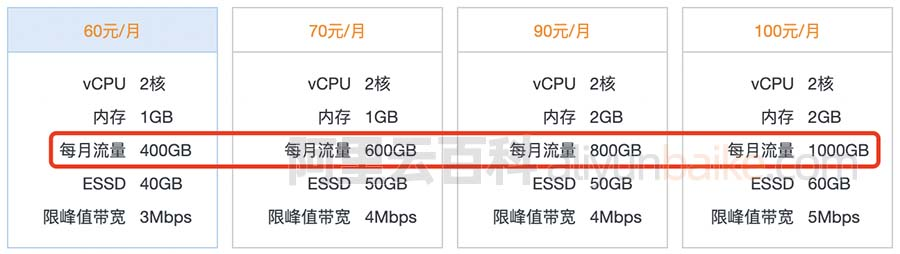0 0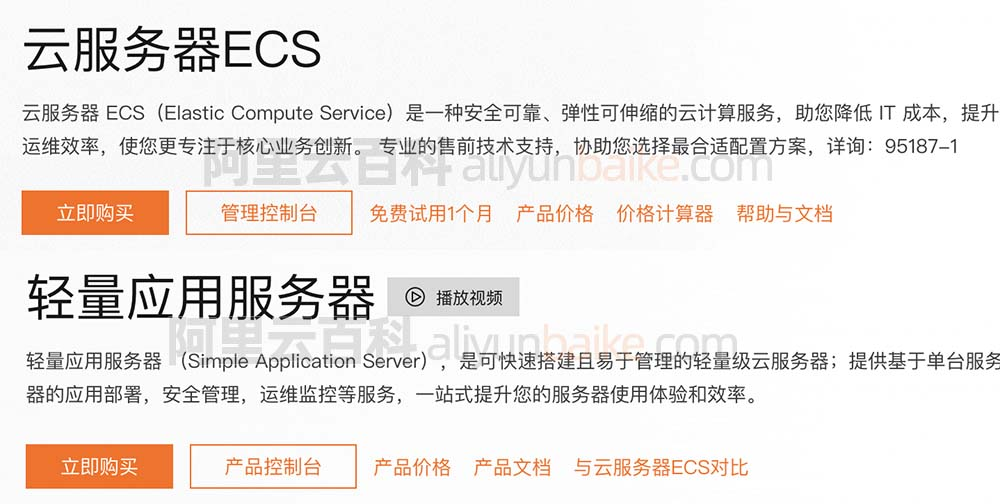0 0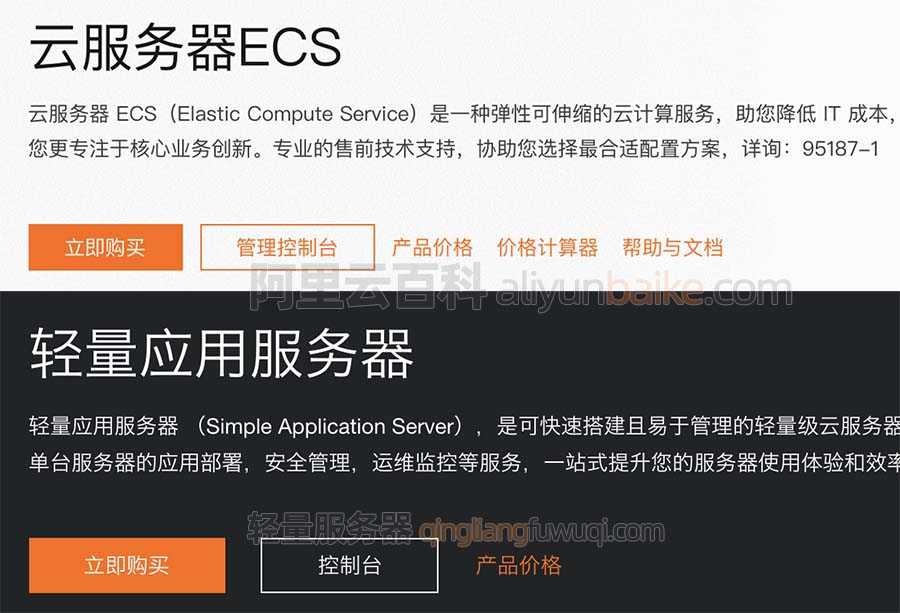0 00 00 0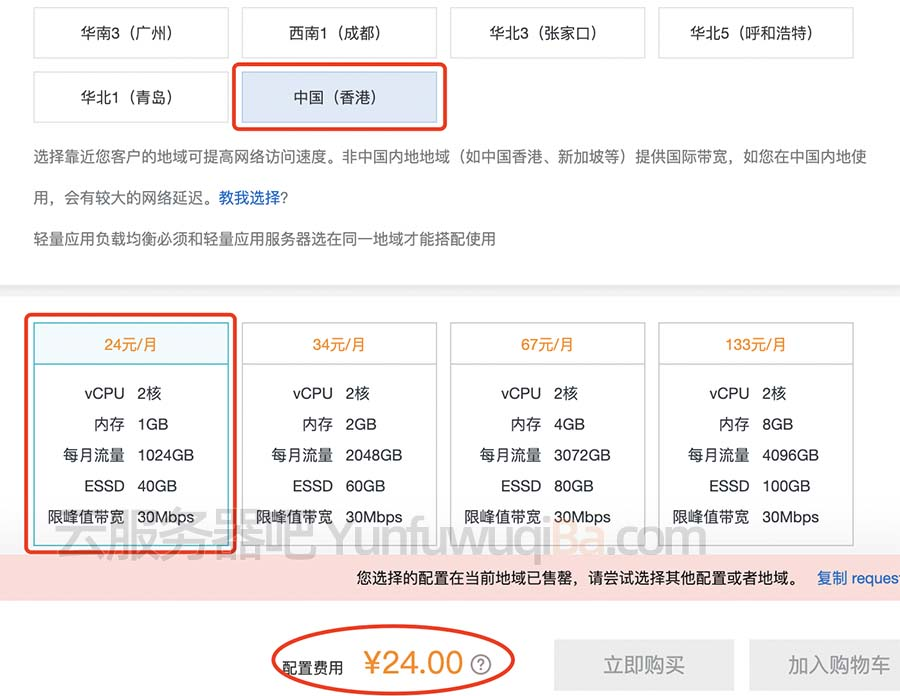0 00 00 0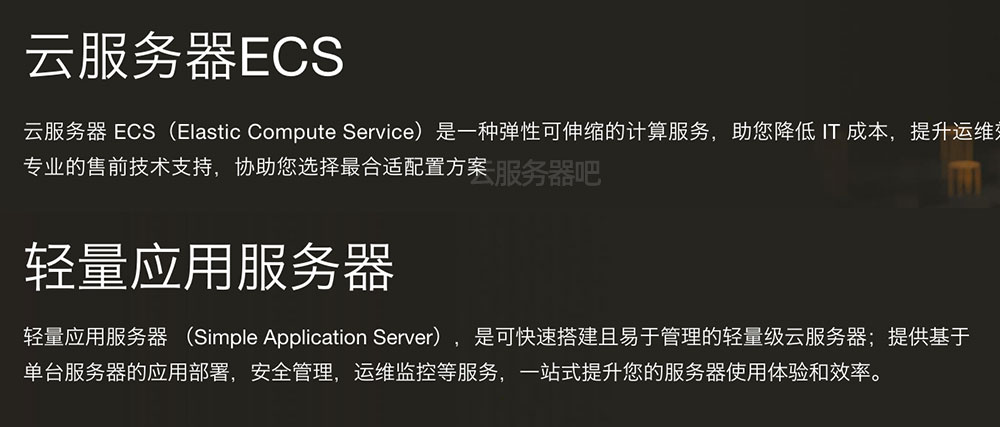0 026913

Clouder认证课程 - 云服务器基础运维与管理765140511

7天玩转云服务器20156092300715430

ECS弹性裸金属服务器产品（神龙）深度解析